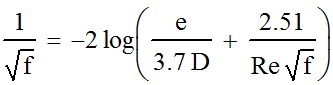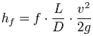# Fluid Mechanics – Basic calculations fluid transport

### BASIC CALCULATION FLOW OF ENERGY REQUIRED, For conveying a fluid through a conduit PRESSURE.

Transporting a fluid through a conduit having sufficient energy required to overcome the friction losses arising in their movement, and the point of discharge flow, and pressure required height.

Inherent properties of the fluid, Dynamic Viscosity (?), Density (?), and the Conditions of Carriage; Laminar regimental (Reynolds No. / Re) (Re < 2000), Turbulent (Re > 4000); conduit characteristics, Length (The), Bore (D), and absolute roughness (?), a decisive influence on the calculation of this value.

The Flow (Q = m3/s), associated with the inner cross section, we determined the average speed (v = m / s), with the fluid to be transported.

The type of pipe used and the constituent material, informs us of the absolute roughness, conduit or internal imperfections, with which the fluid is in its displacement. The layer closest to this internal surface, called Boundary Layer, arise is where the greatest shear, gradient due to a higher speed and thus higher losses.

In most cases, speed values ​​lead to higher Reynolds numbers, with highly turbulent transport regimes, where the values ​​of the friction coefficients (f) predominantly determined relative roughness. The calculation is done with him Coolebrok-White equation, or using the Moody diagram.Expression Coolebrok-White

Retrieved Coefficient of Friction; expression in Darcy-Weisbach, we can calculate the pressure drop (hp = m) column in fluid meters (Julios/Newton). Its meaning is related to the amount of energy we lose (J) per unit weight of fluid (N) we transport in the duct.Expression of Darcy-Weisbach

If we multiply this value by the acceleration of gravity (g = m/s2), The value obtained represents the lost energy (J) transported per unit mass (kg). Ep = J/kg

Knowing the flow rate and in turn the mass flow (m = ?Q) (Kg/s), if we make the product of this value, energy per unit mass loss (hf.g) , find the energy we need to provide the fluid per unit time (Energy Flow), to overcome the losses in transport, and achieve both the pressure drop, at the height of the discharge section.

Conclusion: To ensure this flow of energy, conduction would install a pump that provides steady power to the fluid is transported. Wp (J/s = wattios).

## Did you like this article? Subscribe and you will be updated with all blog articles, CLICK AQUí.Jose Antonio Baron. Autor a Black Hole Innovation. Industrial Engineering Specialty: Electricity, from the Polytechnic University of Catalonia. Industrial Engineer Chemistry from the Polytechnic Branch of Zaragoza. Third Cycle Doctorate and Research Aptitude by University of Valladolid in Fluid Mechanics. Master Business Administration M.B.A. by European Business School will.